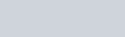Lorem ipsum dolor sit amet, consectetur adipiscing elit, sed do eiusmod tempor incididunt ut labore.

15 St Margarets, NY 10033
(+381) 11 123 4567
ouroffice@aware.com

### KMF#### Using an Array as Function Parameter in JavaScript

In Javascript, we often have data stored as arrays, and functions we want to call. Sometimes, the data in our arrays is exactly the data we want to pass to a function. Fortunately, there are ways in Javascript to use arrays as the input values for functions. Let’s look at how to use arrays as function parameters.

## How to Use Arrays as Function Parameters

When we have a function we want to pass an array to, the most basic way to do it would be like this:

``````let numbers = [ 1, 2, 3 ]
let myFunction = (x, y, z) => {
return x + y + z;
}

// Returns 6
let getCalculation = myFunction(numbers, numbers, numbers);``````

Of course, this can be quite annoying, especially when working with functions that have very long lists of properties. As such, Javascript provides us with two ways to use arrays as function parameters in Javascript – `apply()` and the spread operator.

## Passing Arrays to Functions With the Spread Operator

The modern, and easiest way to pass an array to a function is to use the spread operator. The spread operator (`...`) is simply added before the array. Using our previous example, it looks like this:

``````let numbers = [ 1, 2, 3 ]
let myFunction = (x, y, z) => {
return x + y + z;
}

// Returns 6
let getCalculation = myFunction(...numbers);``````

We can also directly add the array into the function, without needing a variable. For example:

``````let myFunction = (x, y, z) => {
return x + y + z;
}

// Returns 6
let getCalculation = myFunction(...[ 1, 2, 3 ]);``````

If you want to learn everything the spread operator can do, check out my guide on that here.

## Passing Arrays to Functions Using Apply()

The other way to do this is to use `apply()`. If you’re unfamiliar with the main use case of `apply()`, check out my full guide on Javascript’s this object here.

Ultimately, `apply()` lets us take a function, and pass an array into it. The first attribute of `apply()` actually refers to the `this` object we want to use on the function. The second argument is an array of all the parameters for that function. That means you can define the structure of `this` as well as pass an array into the function itself.

This obviously has some specific benefits when compared to the spread (`...`) operator, so it may be more suitable depending on what you want to achieve. Using our previous example, we can pass an array into our function like so:

``````let myFunction = (x, y, z) => {
return x + y + z;
}

// Returns 6
let getCalculation = myFunction.apply({}, [ 1, 2, 3 ]);``````

Here, I am leaving the `this` object empty, so if we did use `this` in `myFunction`, it’d be an empty object – that’s what the first argument of `apply()` does. The second is our array `[1, 2, 3]`, referring to `x`, `y`, and `z` respectively.